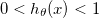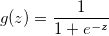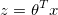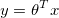## 分类（logistic regression）

``````1. 是本类的：输出为1
2. 非本类的：输出为0
``````

### Binary classificationSigmoid function:``````z = -1000 : 1000;
g = 1 / (1 + exp(-z));
plot(z, g);
``````

hypothesis function``````h(theta, x) = g(theta' * x)
z = theta' * x
g(z) =  1 / (1 + exp(-z))
``````

• 当z >= 0时，g >= 0.5，可以认为输出为1
• 当z < 0时，g < 0.5, 可以认为输出为0

### Decision boundary

• 当theta’ * x >=0时，y = 1
• 当theta’ * x < 时，y = 0``````y = theta' * x
``````

** 这里注意的是，这个边界不一定就是一个直线，虽然是用直线的表达式表述的，但是不要忘了x可以是polynomial. **